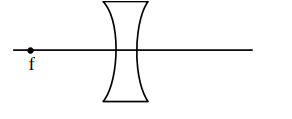# An object is placed at the focus of concave lens having`
Question:

An object is placed at the focus of concave lens having focal length $f$. What is the magnification and distance of the image from the optical centre of the lens?

1. $1, \infty$

2. Very high, $\infty$

3. $\frac{1}{2}, \frac{f}{2}$

4. $\frac{1}{4}, \frac{f}{4}$

Correct Option: , 3

Solution:$U=-f$

$\frac{1}{V}-\frac{1}{U}=\frac{1}{-f} \Rightarrow \frac{1}{V}=-\frac{2}{f}$

$V=\frac{-f}{2}$

$\mathrm{m}=\frac{\mathrm{V}}{\mathrm{U}}=\frac{1}{2}$

distance $=\frac{f}{2}$

Option (3)